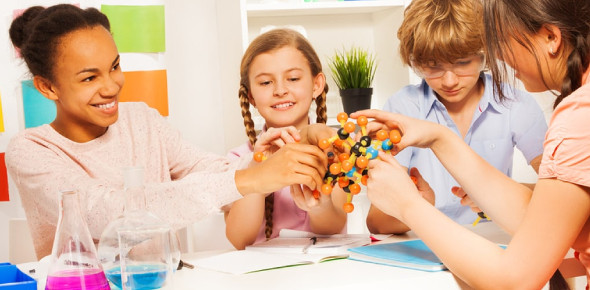# The Ultimate Physical Science Practice Quiz!

151 Questions | Total Attempts: 9135SettingsHey there, are you a student studying Physical science? Or a professional just having fun to recap what he's learned so far? Well, whatever, just sit back, relax and have fun with this Physical Science Practice Quiz, it's going to be quite long.

• 1.
Which of the following is not included in physical science?
• A.

Physics

• B.

Chemistry

• C.

Astronomy

• D.

Zoology

• 2.
What science deals most with energy and forces?
• A.

Biology

• B.

Physics

• C.

Botany

• D.

Agriculture

• 3.
Using superconductors to build computers is an example of
• A.

Technology.

• B.

Applied biology.

• C.

Pure science.

• D.

An experiment.

• 4.
A balance is a scientific tool used to measure
• A.

Temperature.

• B.

Time.

• C.

Volume.

• D.

Mass.

• 5.
Which of the following units is an SI base unit?
• A.

Centimeter

• B.

Kilogram

• C.

Cubic meter

• D.

Liter

• 6.
The composition of the mixture of gases that makes up our air is best represented on what kind of graph?
• A.

Line graph

• B.

Variable graph

• C.

Bar graph

• D.

Pie chart

• 7.
In a controlled experiment,
• A.

The outcome is controlled.

• B.

Results are obtained by computer models.

• C.

One variable is fixed while all others are changed.

• D.

One variable is changed while all others remain fixed.

• 8.
Matter is
• A.

Any visible solid that has mass.

• B.

Any liquid that takes up space and has mass.

• C.

Anything that takes up space and has mass.

• D.

Any liquid or solid that takes up space.

• 9.
What i the chemical formula for iron (III) oxide?
• A.

Fe2+

• B.

NaCl

• C.

I2

• D.

Fe2O3

• 10.
Which of the following is a mixture?
• A.

Air

• B.

Salt

• C.

Water

• D.

Sulfur

• 11.
Compounds and elements are
• A.

Always solids.

• B.

Mixtures.

• C.

Pure substances.

• D.

Dense.

• 12.
Which of the following is a physical change?
• A.

Melting ice cubes

• B.

Burning paper

• C.

Rusting iron

• D.

Burning gasoline

• 13.
If you add oil to water and shake the two liquids together, you will form a
• A.

Heterogeneous mixture.

• B.

Homogeneous mixture.

• C.

Miscible liquid.

• D.

Pure substance.

• 14.
Which of the following assumptions is NOT part of the part of the kinetic theory?
• A.

All matter is made up of tiny, invisible particles.

• B.

Particles are smaller at lower temperature.

• C.

Particles move faster at higher temperature.

• D.

The particles are always moving.

• 15.
During which change of state do atoms or molecules become more ordered?
• A.

Boiling

• B.

Condensation

• C.

Melting

• D.

Sublimation

• 16.
Which of the following describes what happens as the temperature of a gas in a balloon increases?
• A.

The volume of the gas increases and the speed of the particles in increases.

• B.

The speed of the particles decreases.

• C.

The volume decreases.

• D.

The pressure decreases.

• 17.
Fluid pressure is always directed
• A.

Up.

• B.

Down.

• C.

Sideways.

• D.

In all direction.

• 18.
Materials that can flow to fit their containers include
• A.

Gases.

• B.

Liquids.

• C.

Both gases and liquids.

• D.

Neither gases or liquids.

• 19.
If an object weighing 50 N displaces a volume of water with a weight of 10 N, what is the buoyant force on the object?
• A.

10 N

• B.

40 N

• C.

50 N

• D.

60 N

• 20.
Which of Dalton's statements about the atom was later proven false?
• A.

Atoms cannot be subdivided.

• B.

Atoms are tiny.

• C.

Atoms of different elements are not identical.

• D.

Atoms join to form molecules.

• 21.
Which statement is NOT true of Bohr's model of the atom?
• A.

The nucleus can be compared to the sun.

• B.

Electrons orbit the nucleus.

• C.

An electron's path is not known exactly.

• D.

Electrons exist in energy levels.

• 22.
According to the moderns model of the atom,
• A.

Moving electrons form an electron cloud.

• B.

Electrons and protons circle neutrons.

• C.

Neutrons have a positive charge.

• D.

The number of protons an atom has varies.

• 23.
If an atom has a mass of 11 amu and contains five electrons, it's atomic number must be
• A.

55.

• B.

16.

• C.

6.

• D.

5.

• 24.
Which statement about atoms of elements in the same group of the periodic table is true?
• A.

They have the same number of protons.

• B.

They have the same mass number.

• C.

They have similar chemical properties.

• D.

They have the same number of total electrons.

• 25.
The majority of elements in the periodic table are
• A.

Nonmetals.

• B.

Conductors.

• C.

Synthetic.

• D.

Noble gases.

Related TopicsBack to top